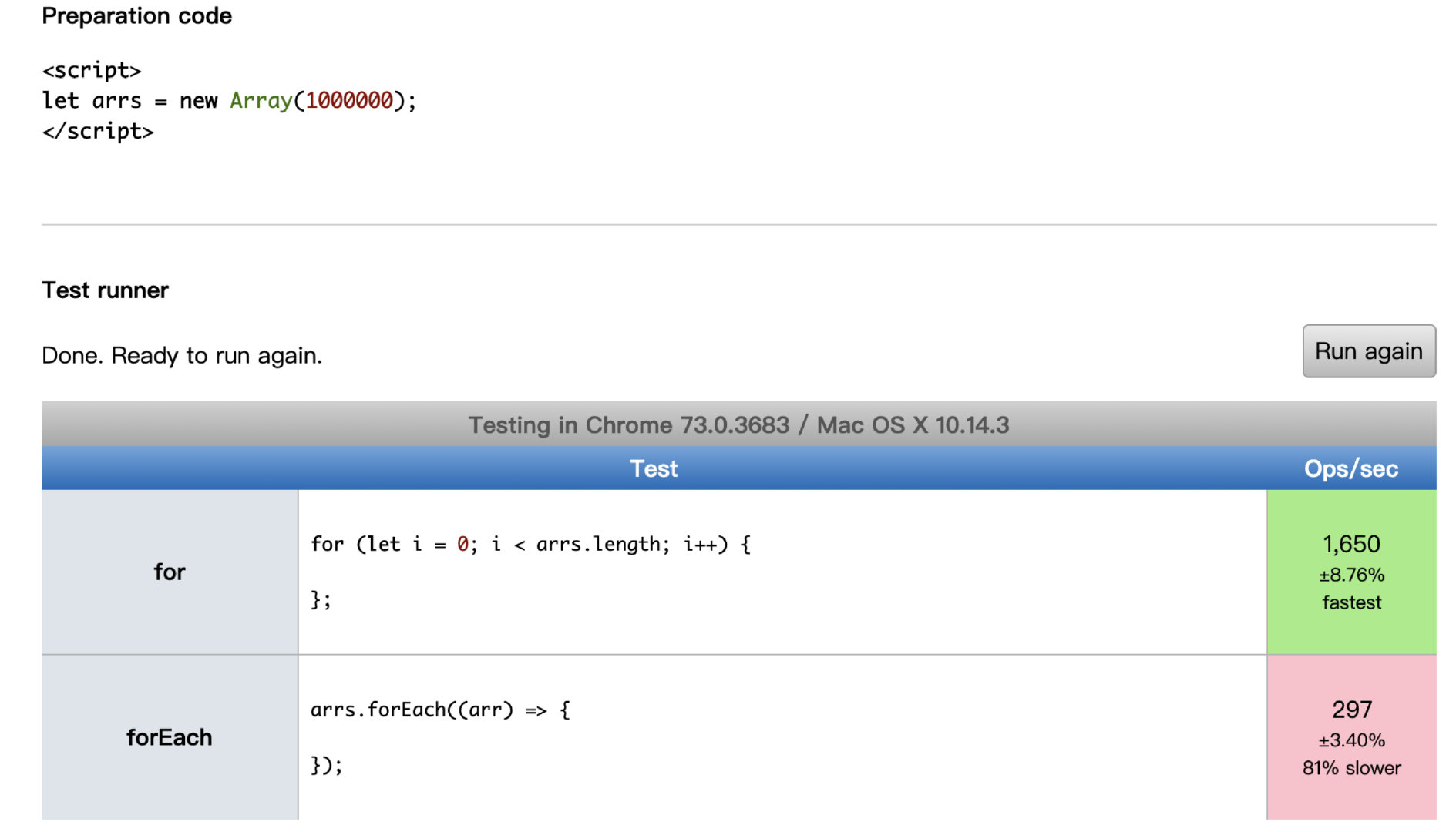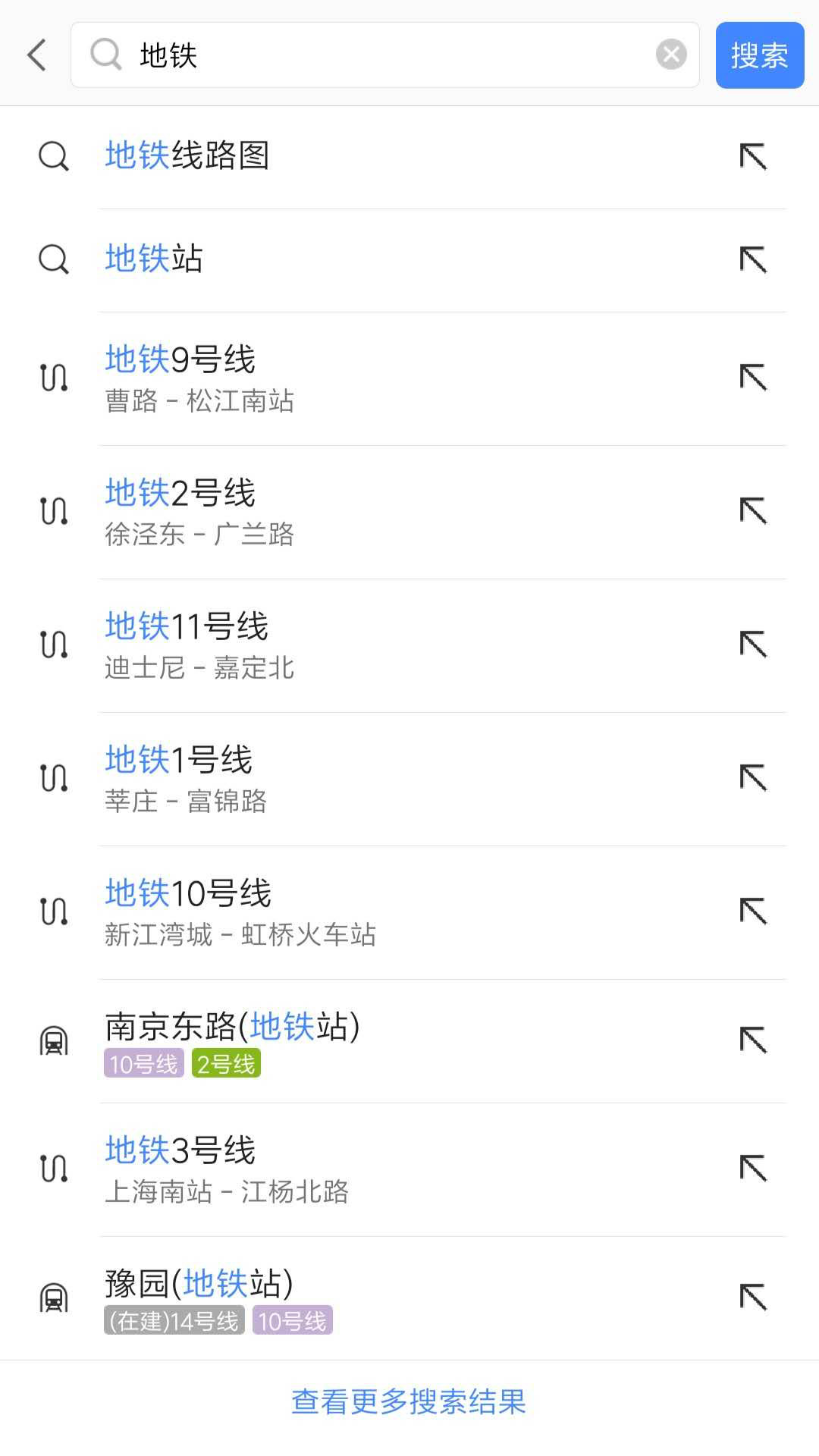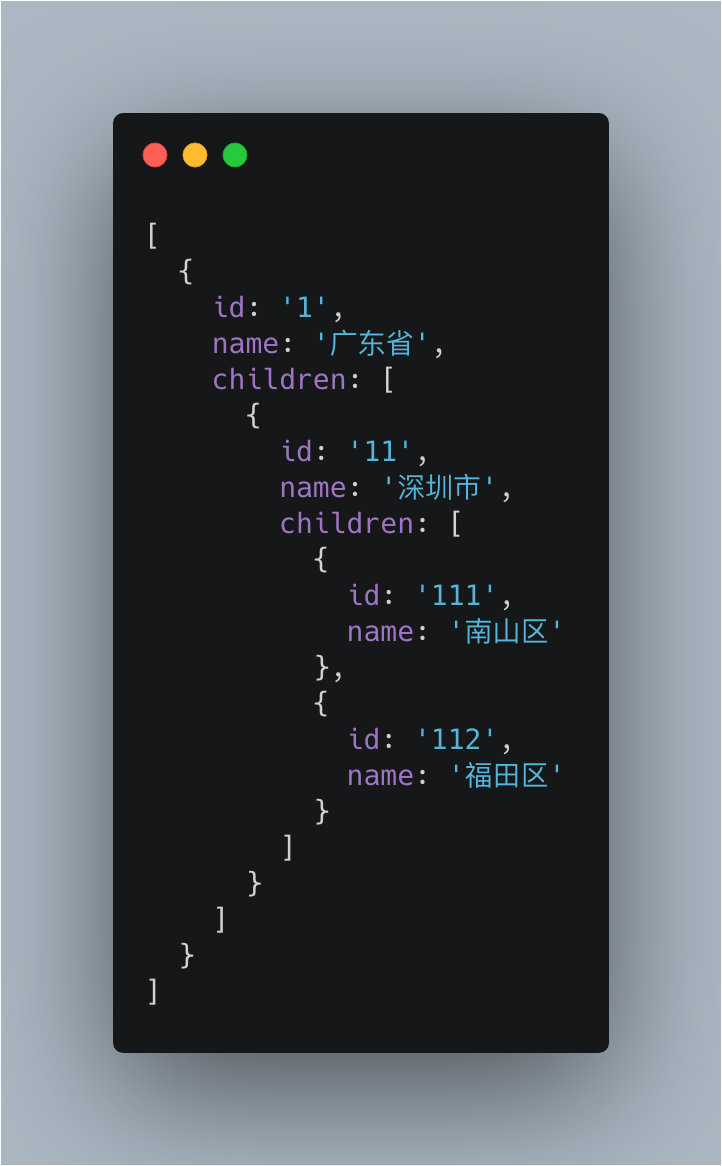# # 壹题汇总

Github 链接在此，欢迎点个 Star### # CSS 世界

``````<img src="1.jpg" style="width:480px!important;”>
``````

### # JS 基础

`Object.prototype.toString.call()``instanceof` 以及 `Array.isArray()`

``````var b = 10;
(function b(){
b = 20;
console.log(b);
})();
``````
``````var b = 10;
(function b(){
b = 20;
console.log(b);
})();
``````
``````var a = 10;
(function () {
console.log(a)
a = 5
console.log(window.a)
var a = 20;
console.log(a)
})()
``````
``````var obj = {
'2': 3,
'3': 4,
'length': 2,
'splice': Array.prototype.splice,
'push': Array.prototype.push
}
obj.push(1)
obj.push(2)
console.log(obj)
``````
``````var a = {n: 1};
var b = a;
a.x = a = {n: 2};

console.log(a.x)
console.log(b.x)
````````````// example 1
var a={}, b='123', c=123;
a[b]='b';
a[c]='c';
console.log(a[b]);

---------------------
// example 2
var a={}, b=Symbol('123'), c=Symbol('123');
a[b]='b';
a[c]='c';
console.log(a[b]);

---------------------
// example 3
var a={}, b={key:'123'}, c={key:'456'};
a[b]='b';
a[c]='c';
console.log(a[b]);
``````
``````function changeObjProperty(o) {
o.siteUrl = "http://www.baidu.com"
o = new Object()
}
let webSite = new Object();
changeObjProperty(webSite);
console.log(webSite.siteUrl);
``````
``````function Foo() {
Foo.a = function() {
console.log(1)
}
this.a = function() {
console.log(2)
}
}
Foo.prototype.a = function() {
console.log(3)
}
Foo.a = function() {
console.log(4)
}
Foo.a();
let obj = new Foo();
obj.a();
Foo.a();
``````
``````String('11') == new String('11');
String('11') === new String('11');
``````
``````var name = 'Tom';
(function() {
if (typeof name == 'undefined') {
var name = 'Jack';
console.log('Goodbye ' + name);
} else {
console.log('Hello ' + name);
}
})();
``````
``````var name = 'Tom';
(function() {
if (typeof name == 'undefined') {
name = 'Jack';
console.log('Goodbye ' + name);
} else {
console.log('Hello ' + name);
}
})();
``````
``````1 + "1"

2 * "2"

[1, 2] + [2, 1]

"a" + + "b"
``````
``````var t1 = new Date().getTime()
for (let i = 0; i < 100; i++) {
for (let j = 0; j < 1000; j++) {
for (let k = 0; k < 10000; k++) {
}
}
}
var t2 = new Date().getTime()
console.log('first time', t2 - t1)

for (let i = 0; i < 10000; i++) {
for (let j = 0; j < 1000; j++) {
for (let k = 0; k < 100; k++) {

}
}
}
var t3 = new Date().getTime()
console.log('two time', t3 - t2)
``````
``````function wait() {
return new Promise(resolve =>
setTimeout(resolve, 10 * 1000)
)
}

async function main() {
console.time();
const x = wait();
const y = wait();
const z = wait();
await x;
await y;
await z;
console.timeEnd();
}
main();
``````
``````function wait() {
return new Promise(resolve =>
setTimeout(resolve, 10 * 1000)
)
}

async function main() {
console.time();
await wait();
await wait();
await wait();
console.timeEnd();
}
main();
``````

### # 异步

``````async function async1() {
console.log('async1 start');
await async2();
console.log('async1 end');
}
async function async2() {
console.log('async2');
}
console.log('script start');
setTimeout(function() {
console.log('setTimeout');
}, 0)
async1();
new Promise(function(resolve) {
console.log('promise1');
resolve();
}).then(function() {
console.log('promise2');
});
console.log('script end');
``````

### # 框架篇

``````class Example extends React.Component {
constructor() {
super();
this.state = {
val: 0
};
}

componentDidMount() {
this.setState({val: this.state.val + 1});
console.log(this.state.val);    // 第 1 次 log

this.setState({val: this.state.val + 1});
console.log(this.state.val);    // 第 2 次 log

setTimeout(() => {
this.setState({val: this.state.val + 1});
console.log(this.state.val);  // 第 3 次 log

this.setState({val: this.state.val + 1});
console.log(this.state.val);  // 第 4 次 log
}, 0);
}

render() {
return null;
}
};
``````

### # 编程题

var arr = [ [1, 2, 2], [3, 4, 5, 5], [6, 7, 8, 9, [11, 12, [12, 13,  ] ] ], 10];

``````for (var i = 0; i< 10; i++){
setTimeout(() => {
console.log(i);
}, 1000)
}
``````
``````var a = ?;
if(a == 1 && a == 2 && a == 3){
console.log(1);
}
``````

``````LazyMan('Tony');
// Hi I am Tony

LazyMan('Tony').sleep(10).eat('lunch');
// Hi I am Tony
// 等待了10秒...
// I am eating lunch

LazyMan('Tony').eat('lunch').sleep(10).eat('dinner');
// Hi I am Tony
// I am eating lunch
// 等待了10秒...
// I am eating diner

LazyMan('Tony').eat('lunch').eat('dinner').sleepFirst(5).sleep(10).eat('junk food');
// Hi I am Tony
// 等待了5秒...
// I am eating lunch
// I am eating dinner
// 等待了10秒...
// I am eating junk food
``````

``````输入: [1, 2, 3, 4, 5, 6, 7] 和 k = 3

``````

``````输入: [-1, -100, 3, 99] 和 k = 2

``````

``````输入: [0,1,0,3,12]

``````

1. 必须在原数组上操作，不能拷贝额外的数组。
2. 尽量减少操作次数。
``````add(1); 			// 1
``````

``````给定 nums = [2, 7, 11, 15], target = 9

``````

``````// 原始 list 如下
let list =[
{id:1,name:'部门A',parentId:0},
{id:2,name:'部门B',parentId:0},
{id:3,name:'部门C',parentId:1},
{id:4,name:'部门D',parentId:1},
{id:5,name:'部门E',parentId:2},
{id:6,name:'部门F',parentId:3},
{id:7,name:'部门G',parentId:2},
{id:8,name:'部门H',parentId:4}
];
const result = convert(list, ...);

// 转换后的结果如下
let result = [
{
id: 1,
name: '部门A',
parentId: 0,
children: [
{
id: 3,
name: '部门C',
parentId: 1,
children: [
{
id: 6,
name: '部门F',
parentId: 3
}, {
id: 16,
name: '部门L',
parentId: 3
}
]
},
{
id: 4,
name: '部门D',
parentId: 1,
children: [
{
id: 8,
name: '部门H',
parentId: 4
}
]
}
]
},
···
];

````````````const value = '112'
const fn = (value) ={
...
}
fn(value) // 输出 [1， 11， 112]

````````````nums1 = [1, 3]
nums2 = 

``````

``````nums1 = [1, 2]
nums2 = [3, 4]

``````

1、只能修改 `setTimeout``Math.floor(Math.random() * 1000` 的代码

2、不能修改 `Math.floor(Math.random() * 1000`

3、不能使用全局变量

``````function print(n){
setTimeout(() => {
console.log(n);
}, Math.floor(Math.random() * 1000));
}
for(var i = 0; i < 100; i++){
print(i);
}
``````

url有三种情况

``````https://www.xx.cn/api?keyword=&level1=&local_batch_id=&elective=&local_province_id=33
https://www.xx.cn/api?keyword=&level1=&local_batch_id=&elective=800&local_province_id=33
https://www.xx.cn/api?keyword=&level1=&local_batch_id=&elective=800,700&local_province_id=33
``````

``````[] || ['800'] || ['800','700']
``````

``````var entry = {
a: {
b: {
c: {
dd: 'abcdd'
}
},
d: {
},
e: 'ae'
}
}

// 要求转换成如下对象
var output = {
'a.b.c.dd': 'abcdd',
'a.e': 'ae'
}
``````
``````var entry = {
'a.b.c.dd': 'abcdd',
'a.e': 'ae'
}

// 要求转换成如下对象
var output = {
a: {
b: {
c: {
dd: 'abcdd'
}
},
d: {
},
e: 'ae'
}
}
``````
1. 如传入的数组元素为`[123, "meili", "123", "mogu", 123]`，则输出：`[123, "meili", "123", "mogu"]`
2. 如传入的数组元素为`[123, [1, 2, 3], [1, "2", 3], [1, 2, 3], "meili"]`，则输出：`[123, [1, 2, 3], [1, "2", 3], "meili"]`
3. 如传入的数组元素为`[123, {a: 1}, {a: {b: 1}}, {a: "1"}, {a: {b: 1}}, "meili"]`，则输出：`[123, {a: 1}, {a: {b: 1}}, {a: "1"}, "meili"]`
``````'abcaakjbb' => {'a':2,'b':2}
'abbkejsbcccwqaa' => {'c':3}
``````